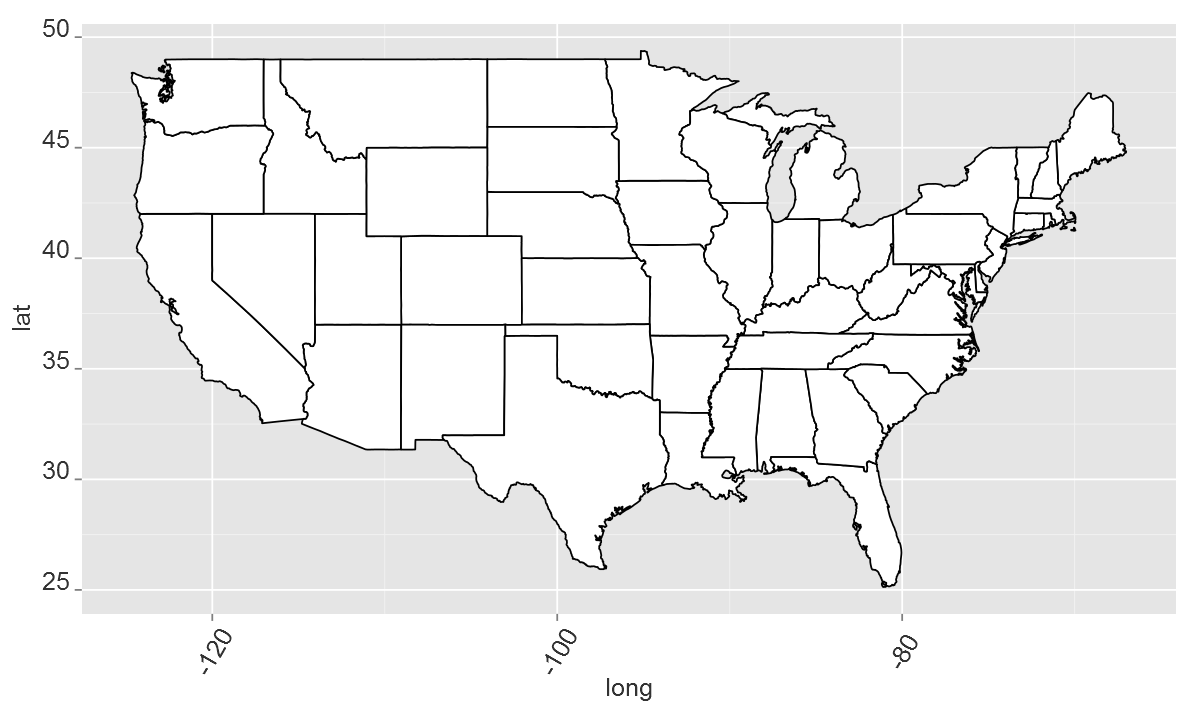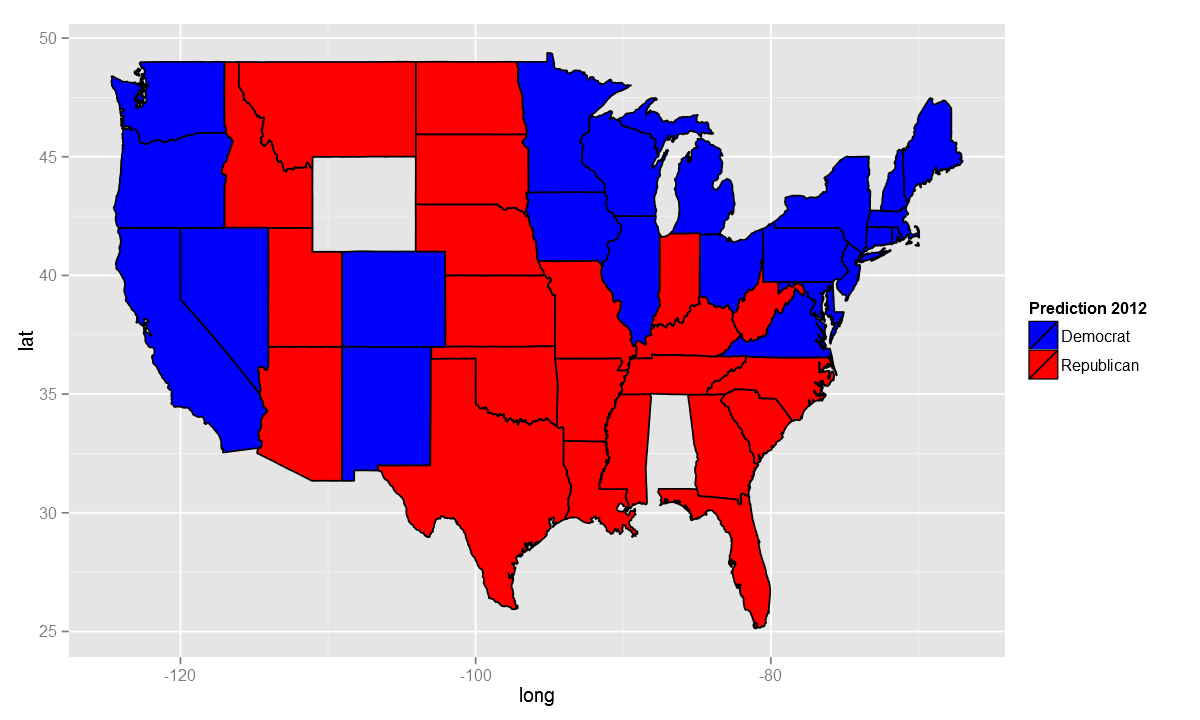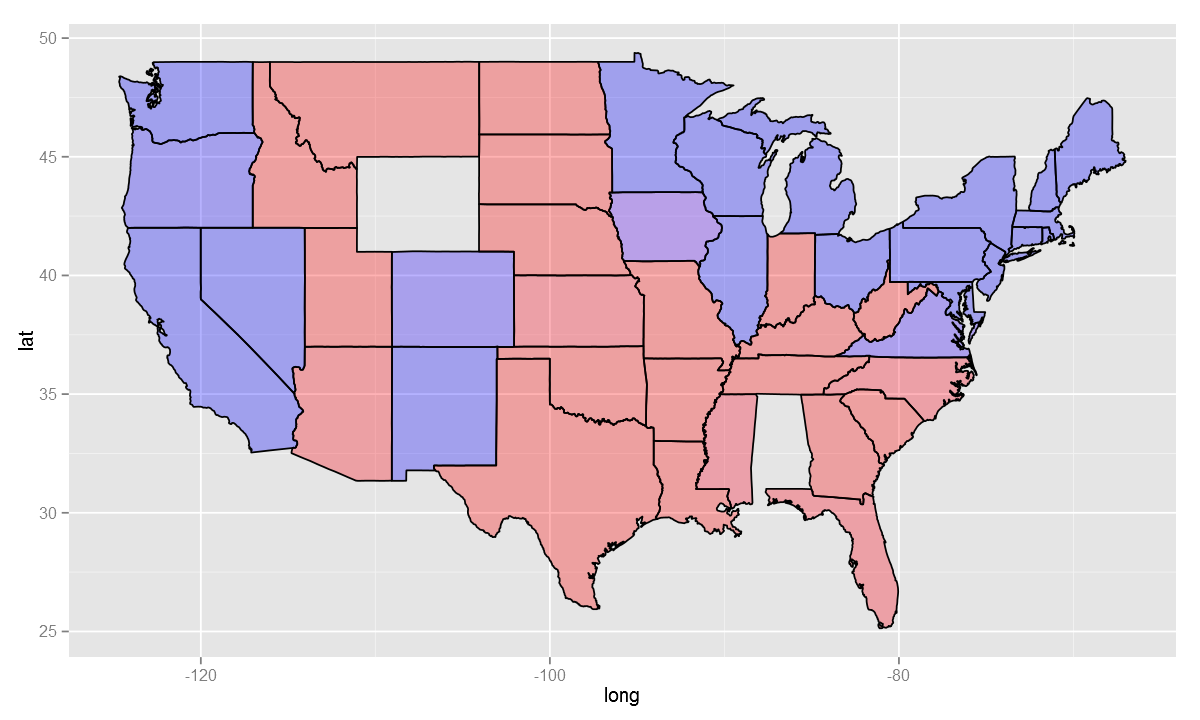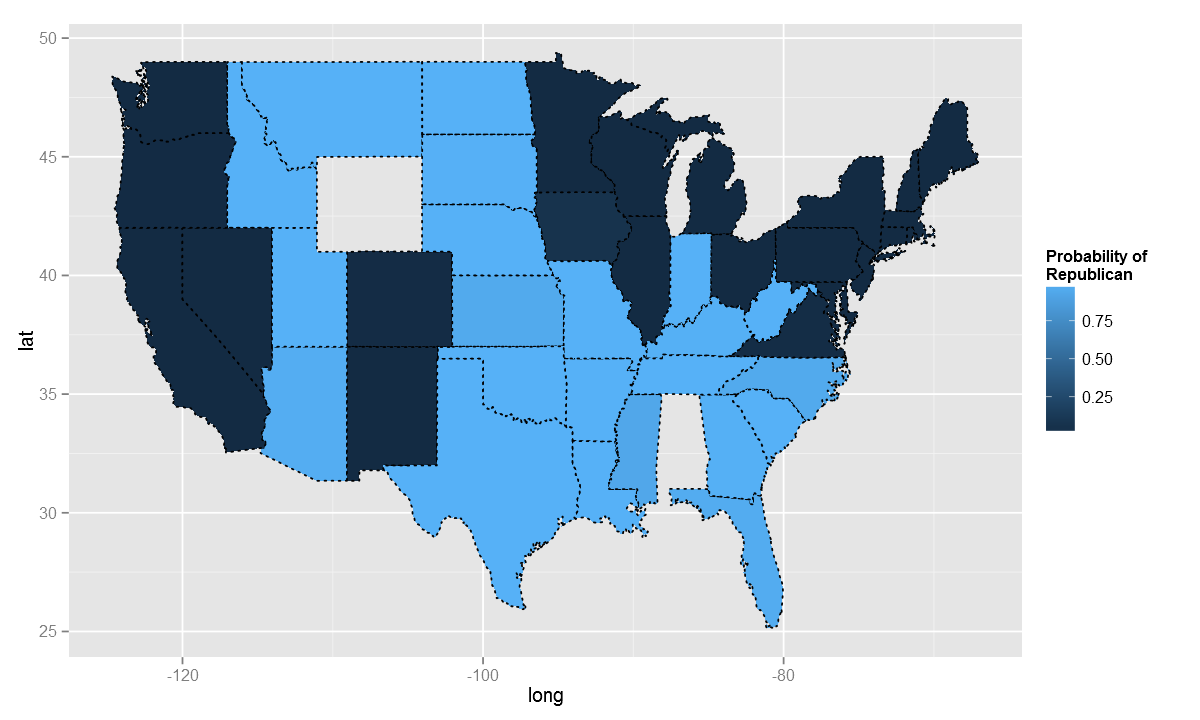# Fisseha Berhane, PhD

#### Data Scientist443-970-2353[email protected] CV Resume## Visualizing election predictions using ggplot2¶

Here, ggplot2 is used to visualize US presidential election predictions using logistic regression on polling data.

First, the data is separated into a training set, containing data from 2004 and 2008 polls, and a test set, containing the data from 2012 polls.Then a logistic regression model is developed to forecast the 2012 US presidential election.

In :
options(jupyter.plot_mimetypes = 'image/png')

options(repr.plot.width = 10)
options(repr.plot.height = 6)

In :
library(ggplot2)
library(maps)
library(ggmap)

statesMap = map_data("state")


We can now plot the states using ggplot2

In :
ggplot(statesMap, aes(x = long, y = lat, group = group)) +
geom_polygon(fill = 'white', color = "black") +
theme(axis.title.y = element_text(colour="grey20",size=15,angle=90,hjust=.5,vjust=1,face="plain"),
axis.title.x = element_text(colour="grey20",size=15,angle=0,hjust=.5,vjust=1,face="plain"),
axis.text.y = element_text(colour="grey20",size=15,angle=0,hjust=1,vjust=0,face="plain"),
axis.text.x = element_text(colour="grey20",size=15,angle=60,hjust=.5,vjust=.5,face="plain"))

Out:Now, let’s develop a logistic regression model and map the prediction results.

In :
url<-"https://courses.edx.org/asset-v1:[email protected]+block/PollingImputed.csv"

# Subset data into training set and test set
Train = subset(polling, Year == 2004 | Year == 2008)
Test = subset(polling, Year == 2012)

mod2 = glm(Republican~SurveyUSA+DiffCount, data=Train, family="binomial")

TestPrediction = predict(mod2, newdata=Test, type="response")


TestPrediction gives the predicted probabilities for each state, but let’s also create a vector of Republican/Democrat predictions

In :
TestPredictionBinary = as.numeric(TestPrediction > 0.5)


To use ggplot2, predictions and state labels have to be in a data frame.

In :
predictionDataFrame = data.frame(TestPrediction, TestPredictionBinary, Test$State)  Now, we need to merge “predictionDataFrame” with the map data “statesMap”. Before doing so, we need to convert the Test.State variable to lowercase, so that it matches the region variable in statesMap. In : predictionDataFrame$region = tolower(predictionDataFrame$Test.State) predictionMap = merge(statesMap, predictionDataFrame, by = "region")  we need to make sure the observations are in order so that the map is drawn properly. In : predictionMap = predictionMap[order(predictionMap$order),]


Now, we can plot the predictions.

In :
ggplot(predictionMap, aes(x = long, y = lat, group = group, fill = TestPredictionBinary))+
geom_polygon(color = "black") + scale_fill_gradient(low = "blue", high = "red", guide = "legend", breaks= c(0,1), labels = c("Democrat", "Republican"), name = "Prediction 2012")

Out:We can play with the color, bourder thickness, line type and transparency.

In :
ggplot(predictionMap, aes(x = long, y = lat, group = group, fill = TestPrediction))+
geom_polygon(color = "black", alpha=0.3) +
scale_fill_gradient(low = "blue", high = "red", guide = "legend", breaks= c(0,1))

Out:We can also plot the probabilities instead of the binary predictions. Using the deafult color:

In :
ggplot(predictionMap, aes(x = long, y = lat,
group = group, fill = TestPrediction,name='Fish'))+
geom_polygon(color = "black",lty=3)+
scale_fill_continuous(name="Probability of\nRepublican")

Out: